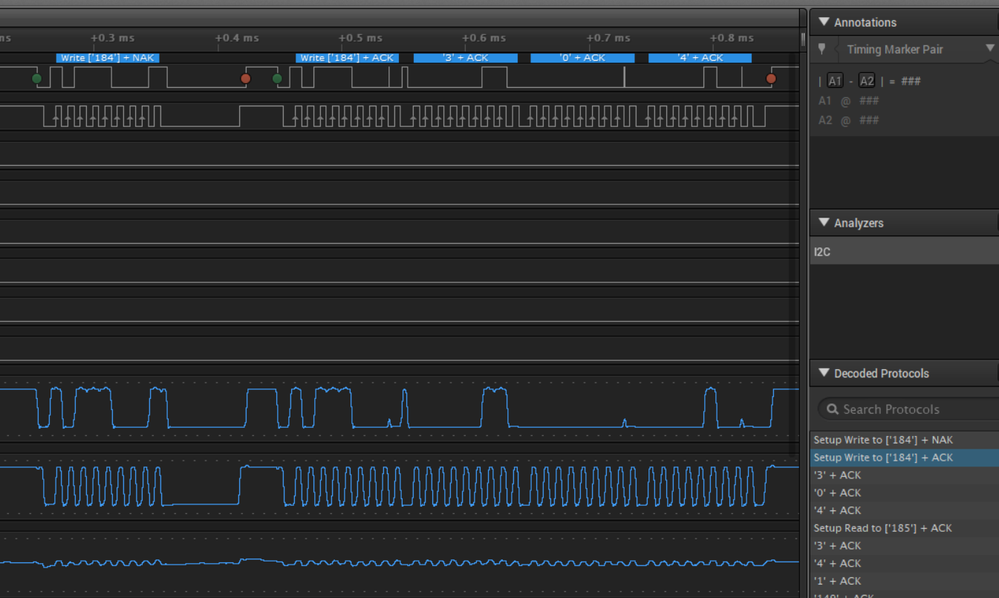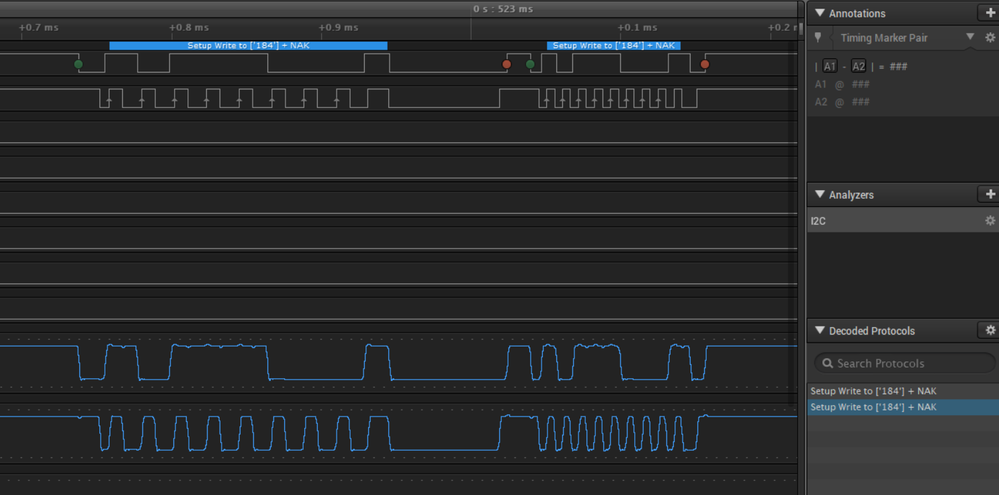## K22 I2C and NACK

cancel
Showing results for
Did you mean:

## K22 I2C and NACK

433 ViewsContributor IV

Greetings!

I am using the K22FN256LH12 microcontroller with KDSv 3.2 and Processor Expert, and I am trying to get it to work with this AM2315 temperature sensor. I am currently using the I2C_LDD to communicate through I2C to other peripherals as well as this one. This sensor keeps giving me issues though. The first image below is of the sensor working correctly on an arduino, and the second is mine. I do not understand why I keep getting a NACK, any suggestions?Here is my code, I had to bit bang the first "184" since it needs a unique delayed stop...

```

byte err;
byte i = 0;

//first thing is to turn on the 5V
V5_ENABLE;

//now bit-bang the wakeup since the I2C h/w cannot do this
//set mux to change I2C to GPIO (MUX=1)
PORTB_PCR1 &= ~(1 << 9);
PORTB_PCR1 |= (1 << 8);
PORTB_PCR0 &= ~(1 << 9);
PORTB_PCR0 |= (1 << 8);

//set data direction register for output
//must be done in following order or glitch will result on output
GPIOB_PDOR |= 0x3; //set BUS IDLE
GPIOB_PDDR |= 0x3;

WAIT1_Waitms(1);

GPIOB_PDOR &= ~(1 << 1); //set SDA 0
WAIT1_Waitus(10);
GPIOB_PDOR &= ~(1 << 0); //SCL is 0
WAIT1_Waitus(1);
//
for (i = 0; i < 8; i++) {
GPIOB_PDOR |= (1 << 1); //set SDA 1
} else {
GPIOB_PDOR &= ~(1 << 1); //set SDA 0
}
WAIT1_Waitus(1); //and clock it out
GPIOB_PDOR |= (1 << 0); //SCL is 1
WAIT1_Waitus(5);
GPIOB_PDOR &= ~(1 << 0); //SCL is 0
WAIT1_Waitus(5);
}
//send ACK
GPIOB_PDOR |= (1 << 1); //set SDA 1
WAIT1_Waitus(1);
GPIOB_PDOR |= (1 << 0); //SCL is 1
WAIT1_Waitus(10);
GPIOB_PDOR &= ~(1 << 0); //SCL is 0
GPIOB_PDOR &= ~(1 << 1); //set SDA 0

//wait a millisecond (required by sensor and not to exceed 3ms)
WAIT1_Waitus(70);

//send STOP
GPIOB_PDOR |= (1 << 0); //set SCL high
WAIT1_Waitus(2);
// GPIOB_PDOR |= (1 << 1); //set SDA high
// }// end of bit bang

//set GPIO pin mux back to I2C (MUX= 0x02)
PORTB_PCR0 |= (1 << 9);
PORTB_PCR0 &= ~(1 << 8);
PORTB_PCR1 |= (1 << 9);
PORTB_PCR1 &= ~(1 << 8);

MyI2CPtr = I2C_Sensors_Init(NULL);
//at this point , can use regular I2C
//I2C_TxCompleted = false;
tx_buf = 0xB8;
tx_buf = 0x3U; //start at register 0
tx_buf = 0; //request four
tx_buf = 0x4U; //request four

uint8_t InpData;
I2C_TxCompleted = FALSE;
err = I2C_Sensors_MasterSendBlock(MyI2CPtr, tx_buf, 4, LDD_I2C_SEND_STOP);
while (!I2C_TxCompleted) { /* Wait until OutData are transmitted */
I2C_Sensors_Main(MyI2CPtr);
}
I2C_TxCompleted = FALSE;
WAIT1_Waitms(2); //wait atleast 1.5ms for sensor to start reply
err = I2C_Sensors_MasterReceiveBlock(MyI2CPtr, InpData, 8U, LDD_I2C_SEND_STOP);
while (!I2C_TxCompleted) { /* Wait until OutData are transmitted */
I2C_Sensors_Main(MyI2CPtr);
}

```

Any ideas would be much appreciated!

Labels (1)
• ### General

0 Replies